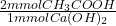## A 5.00 mL sample of vinegar, a solution of acetic acid, is titrated with 0.756 M calcium hydroxide. 10.23 mL of the calcium hydroxide is req

Question

A 5.00 mL sample of vinegar, a solution of acetic acid, is titrated with 0.756 M calcium hydroxide. 10.23 mL of the calcium hydroxide is required to reach the equivalence point. What is the molarity of the acetic acid in the vinegar.

in progress 0
6 months 2021-07-22T02:17:27+00:00 1 Answers 11 views 0

## Answers ( )

3.09 M

Explanation:

The reaction that takes place is:

• 2CH₃COOH + Ca(OH)₂ → 2CH₃COO⁻ + Ca⁺² + 2H₂O

First we calculate how many Ca(OH)₂ moles reacted, using the given volume and concentration:

• 10.23 mL * 0.756 M = 7.73 mmol Ca(OH)₂

Then we convert Ca(OH)₂ mmoles into mmoles of acetic acid, using the stoichiometric coefficients of the balanced reaction:

• 7.73 mmol Ca(OH)₂ *= 15.46 mmol CH₃COOH

Finally we calculate the molarity of acetic acid, using the number of moles and given volume:

• 15.46 mmol / 5.00 mL = 3.09 M